Purva Huilgol — Updated On May 8th, 2020

## Starting your Deep Learning Career?

Deep learning can be a complex and daunting field for newcomers. Concepts like hidden layers, convolutional neural networks, backpropagation keep coming up as you try to grasp deep learning topics.

It’s not easy – especially if you take an unstructured learning path and don’t cover your basic fundamental concepts first. You’ll be stumbling around a foreign city like a tourist without a map!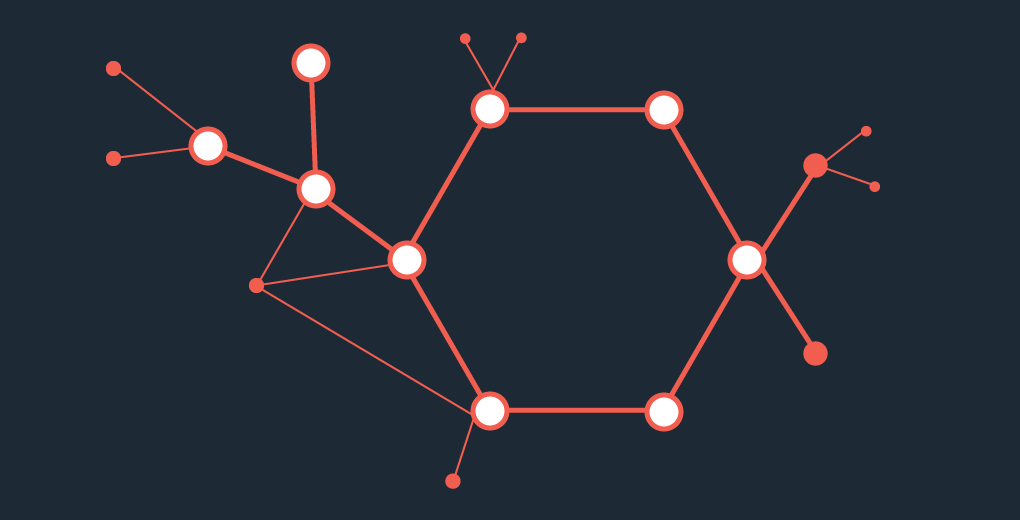Here’s the good news – you don’t need an advanced degree or a Ph.D. to learn and master deep learning. But there are certain key concepts you should know (and be well versed in) before you plunge into the deep learning world.

I’ll be covering five such essential concepts in this article. I also recommend going through the below resources to augment your deep learning experience:

### The five essentials for starting your deep learning journey are:

2. Python programming
3. Linear Algebra and Calculus
4. Probability and Statistics
5. Key Machine Learning Concepts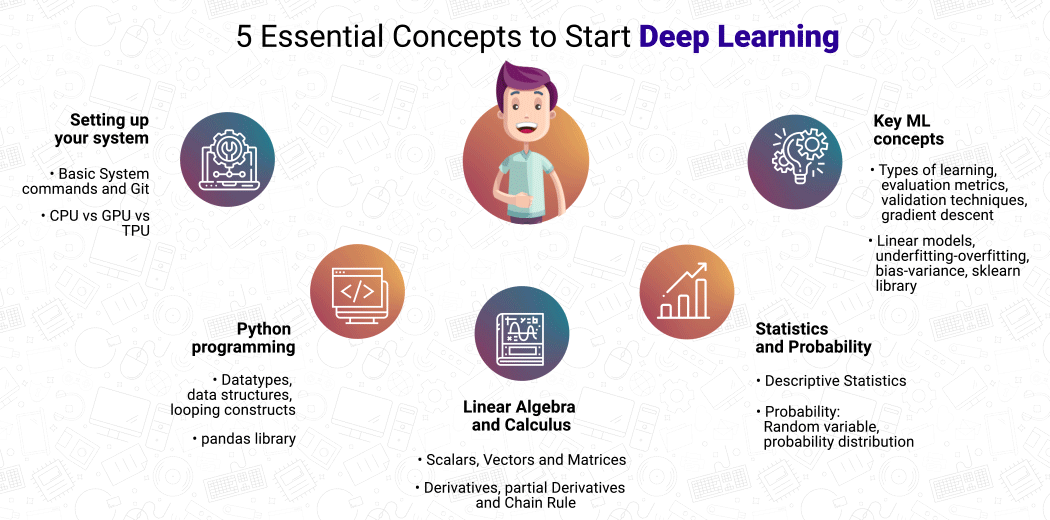Let’s go over them one by one.

For learning a new skill, say cooking, you would first need to have all the equipment. You would need tools like a knife, a cooking pan, and of course, a gas stove! You would also need to know how to use the tools given to you.Similarly, it is important to set up your system for deep learning, have some knowledge of the tools you would need, and how to use them.

Regardless of your operating system, Windows, Linux or Mac, it is important to know the basic commands. Here is a handy table for your reference: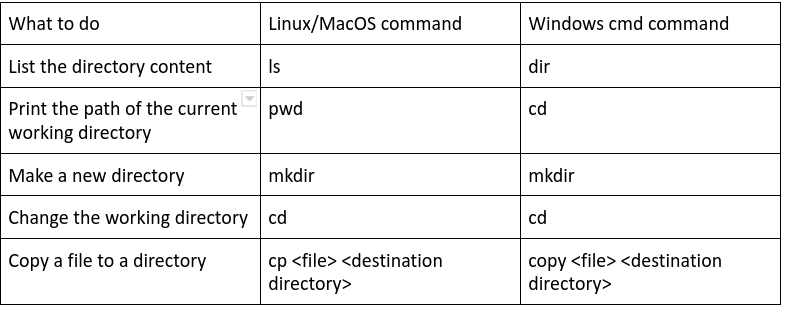Here is a great tutorial to get started with Git and the basic Git commands: Git – Tutorial.

The Deep learning boom has not only brought path-breaking research in the field of AI but has also broken new barriers in computer hardware.

### GPU (Graphics Processing Unit):

You would need a GPU to work with image and video data for most deep learning projects. You can build a deep learning model on your laptop/PC without the GPU as well, but then it would be extremely time-consuming to do. The main advantages a GPU has to offer are:

1. It allows for parallel processing
2. In a CPU+GPU combination, CPU assigns complex tasks to GPU and other tasks to itself, thereby saving a lot of time

Here is a great video explaining the difference between a GPU and CPU:

The best part? You don’t need to buy a GPU or get one installed on your machine. There are multiple Cloud Computing resources that provide GPUs either for free or for an extremely low cost. Additionally, there are a few GPUs that come preinstalled with some practice datasets and their own tutorials preloaded. Some of them are Paperspace Gradient, Google Colab, and Kaggle Kernels.

On the other hand, there are full-fledged servers as well which require some installation steps and some customization like Amazon Web Services EC2.

Here is a table illustrating the options you have: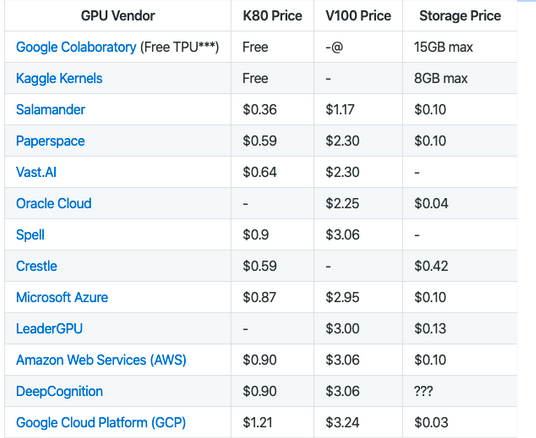Deep Learning has also led to Google developing its own type of Processing Units exclusively catering towards building neural networks and deep learning tasks – TPUs.

### TPUs

TPU, or Tensor Processing Unit, is essentially a co-processor which you use with the CPU. Cheaper than a GPU, a TPU is much faster and thus makes building deep learning models affordable.

Google Colab also provides free usage of the TPU (not its full-fledged enterprise version, but a cloud version). Here is Google’s own Colab tutorial on working with TPUs and building models on them: Colab notebooks | Cloud TPU.

To summarize, here are the basic minimum hardware requirements to start cooking your deep learning model: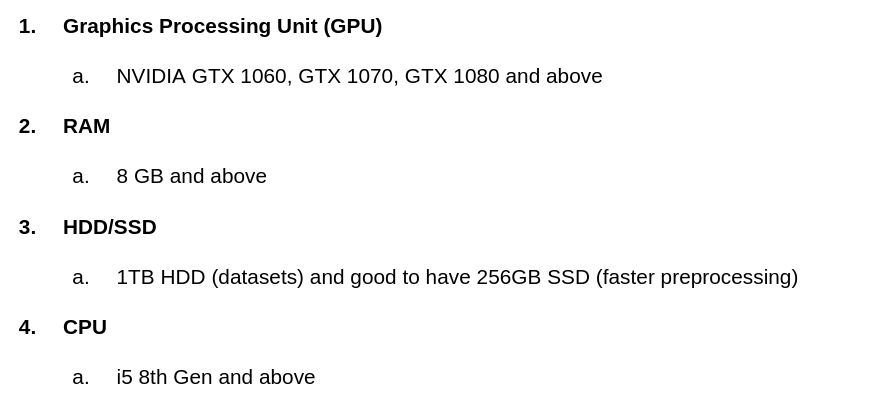## 2. Python Programming

Continuing the same analogy of learning to cook, you have now got the hang of operating a knife and a gas stove. But what about the skills and the recipes needed to actually cook food?

This is where we encounter the software required for deep learning. Python is a programming language that is used across industries for deep learning.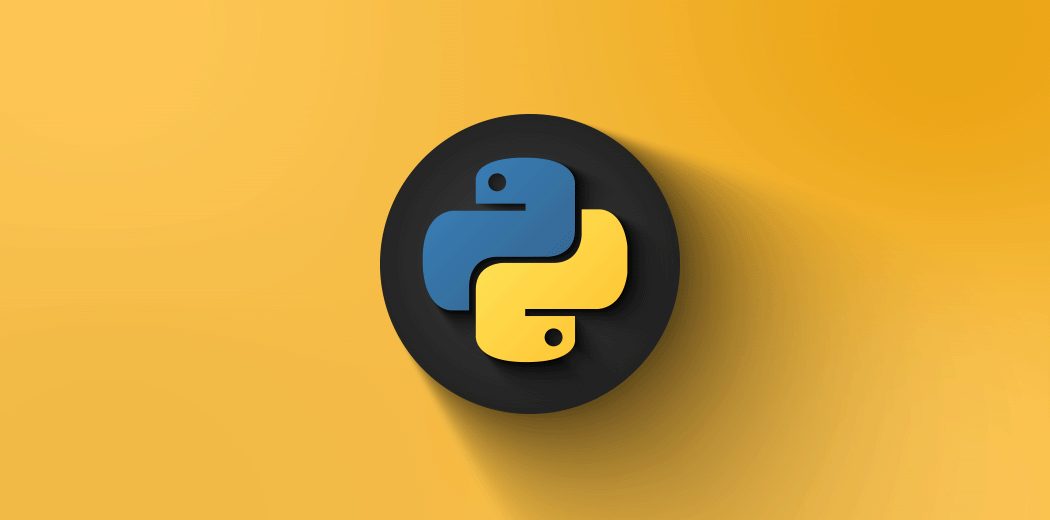However, we can’t use only Python for the level of computations and operations that deep learning needs. Additional functionalities are provided by what are known as libraries in Python. A library can have hundreds of small tools, called functions, that we can use for programming.

• While you don’t need to be a coding ninja for Deep Learning, you do need to know the basics of programming in Python
• That being said, rather than mastering the vast ocean that is programming in Python, you can start off by learning about some specific libraries exclusively geared towards machine learning and dealing with data

Anaconda is a framework that helps you keep track of your Python versions and the libraries as well. It is a handy all-in-one tool that is quite popular, easy to work with, and has simple documentation as well. Here is how you can install Anaconda.

So what do I mean by the basics of Python? Let’s discuss this in a bit more detail.

Note: You can start learning Python in our free and popular course – Python for Data Science.

### 1. Variables and data types in Python

The main data types in Python are:

• Int: Integer numbers, can be signed
• Float: Decimal numbers
• String: a single character or a sequence of characters
• Bool: to hold the 2 boolean values – True and False

### 2. Operators in Python

There are 5 main types of operators in Python:

• Arithmetic operators: Like +, -, *, /, etc
• Comparison operators: like <, >, <=, >=, ==, !=
• Logical operators: and, or, not
• Identity operators: is, is not
• Membership operators: in, not in

### 3. Data Structures in Python

Python offers a variety of datasets that we can use for different purposes. Each data structure has its unique properties that we can leverage to store different types of data and data types. These properties are:

• Ordered: This means that there is a specific order in which the elements in the data structure are stored. No matter how and when we use it, this order remains the same (unless we change it explicitly)
• Immutable: This means that the data structure cannot be changed. If a data structure is mutable, it means that it can be changed

In data science, the most frequently used data structures are:

• Lists: Ordered and mutable

Example: We have a list like this:

`my_list = [1, 3, 7, 9]`

This order will remain the same everywhere we use this list. Also, we can change this list, like removing 7, adding 11, etc.

• Tuple: Similar to a list (it is ordered), but unlike a list, a tuple is immutable

Example: A tuple can be declared as:

`my_tuple = ("apple", "banana", "cherry") `

Now, again, this order will remain the same, but unlike a list, we cannot remove ‘cherry’, or add ‘orange’ to the tuple.

• Sets: Unordered and mutable, though they can only hold unique values

Example: A set uses curly braces like this:

` my_set = {'apple', 'banana', 'cherry'}`

The order is not defined for a set.

• Dictionaries: A set of <key, value> pairs. A dictionary is unordered and changeable. This means that they essentially have no order, can be changed, but yet can be accessed via indices or keys. A dictionary can have only unique keys, though a key may not necessarily hold a unique value.

Example: A dictionary also uses curly braces with a key-value format:

`my_dict = { "brand": "Ford",  "model": "Mustang",  "year": 1964}`

Here, ‘brand’, ‘model’, and ‘year’ are the keys that have the values ‘Ford’, ‘Mustang’, and ‘1964’ respectively. The order of the keys can be different every time you print the dictionary.

### 4. Control Flow in Python

Control flow means controlling the flow of the execution of your code. We execute the code line by line, and what we execute on a line affects how we write the next line of code: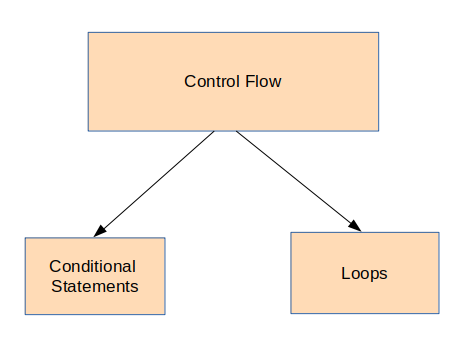#### Conditional statements

These are used to set a condition with the conditional operators we saw earlier.

• if-else: What would you like to eat today? A burger, or a salad? If you want the healthier option, you would go for the salad, or if you just wanted a quick bite without caring for the calories, you would choose the burger. That is what the if-else conditional statement does

Example: You need to check if a student has passed or failed. If he has obtained marks >= 40, he has passed, otherwise, he has failed.

In that case, our conditional statement would be:

```if marks >= 40:
print("Pass")
else:
print("Fail")```

#### Loops

• The for loop: Used to iterate over a sequence. The sequence can mean a sequence of characters (a string) or any of the data structures above like lists, sets, tuples, and dictionaries

Example: We have a list having values from 1 to 5 and we need to multiply each value in this list with 3:

```numbers_list = [1, 2, 3, 4, 5]

for each_number is numbers_list:
print(each_number * 3)```

Try out the above snippets and you can see how easy Python is!

Fun note: Unlike other programming languages, we don’t need to store variables of the same type in a data structure. We can totally have a list like this [John, 153, 78.5, “A+”] or even a list of lists like [[“A”, 56], [“B”, 36.5]]. It is this variety and flexibility of Python that has made it so popular among data scientists!

You can also avail the below free courses that cover Python and Pandas essentials:

### 5. Pandas Python

This is one of the first libraries you would come across when you start Machine Learning and Deep Learning. An extremely popular library, Pandas is just as required for deep learning as for machine learning.

We store data in a variety of formats, such as CSV (Comma Separated Values) file, Excel sheets, etc. In order to work with the data in these files, Pandas provides a data structure called a Pandas dataframe (you can think of it as a table).

Dataframes and the sheer number of manipulation operations Pandas provides on dataframes make it the workhorse library for machine and deep learning.

You can take this free and easy course to get started with Pandas if you haven’t already: Pandas for Data Analysis in Python.

Now, if you read the list of 5 things we started out with, you might have a question: What do we do with all the mathematics in deep learning?

Well, let’s find out!

## 3. Linear Algebra and Calculus for Deep Learning

There is a common myth that Deep Learning requires advanced knowledge of linear algebra and calculus. Well, let me dispel that myth right here.

You only need to recollect your high school-level math to start your Deep Learning journey!

Let us take a simple example. We have images of cats and dogs and we want the machine to tell us which animal is present in any given image: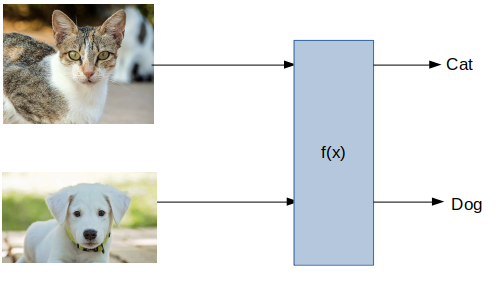Now, we can easily identify the cat and the dog here. But how will the machine distinguish the two? The only way is to give this data to the model in the form of numbers, and that is where we need linear algebra. We basically convert the images of a cat and a dog into numbers. These numbers can be either expressed as vectors or as matrices.

We will cover some key terms and some great resources you can learn from.

### Linear Algebra for Deep Learning

1. Scalars and vectors: While scalars only have magnitude, vectors have both direction and magnitude.

• Dot product: The Dot product of 2 vectors returns a scalar value
• Cross product: The Cross product of two vectors returns another vector which is orthogonal (right-angled) to both

Example: If we have 2 vectors a = [1, -3, 5] and b = [4, -2, -1], then:

a) Dot product:

`a . b = (a1 * b1) + (a2 * b2) + (a3 * b3) = (1 * 4) + (-3 * -2) + (5 * 1) = 3`

b) Cross product:

`a X b = [c1, c2, c3] = [13, 21, 10]`

where,

```c1 = (a2*b3) - (a3*b2)
c2 = (a3*b1) - (a1*b3)
c3 = (a1*b2) - (a2*b1)```

2. Matrices and Matrix Operations: A matrix is an array of numbers in the form of rows and columns. Now, for example, the above image of a cat can be written as a matrix of pixels: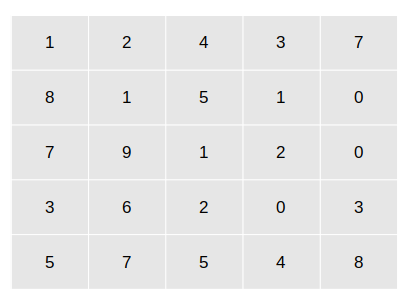Just like numbers, we can perform operations like adding and subtracting two matrices. However, operations like multiplication and division are performed slightly differently from the regular way:

• Scalar Multiplication: When we multiply a single scalar value with a matrix, we multiply the scalar with all the elements in the matrix
• Matrix Multiplication: Multiplying 2 matrices means calculating the dot product of the rows and columns and creating a new matrix with different dimensions than the 2 input matrices
• Transpose of the matrix: We swap the rows and the columns in a matrix to get its transpose
• Inverse to the matrix: Conceptually similar to inverting numbers, an inverse of a matrix multiplied with the matrix gives you an identity matrix

You can refer to this excellent Khan Academy course on Linear Algebra to learn the above concepts in detail. You can also check out 10 powerful applications of linear algebra here.

### Calculus for Deep Learning

The value we are trying to predict, say, ‘y’, is whether the image is a cat or a dog. This value can be expressed as a function of the input variables/input vectors. Our main aim is to make this predicted value as close to the actual value.

Now, imagine dealing with thousands of cat images and dog images. These are surely cute to look at, but you can imagine that working on these images and numbers is not easy at all!

Since deep learning essentially involves large amounts of data and complex machine learning models, working with both is often time and resource expensive. That is why it is important to optimize our deep learning model in such a way that it is able to predict as accurately as possible without using too many resources and time.

This is where the crux of the calculus used in deep learning lies: Optimization.

In any deep learning or machine learning model, we can express the output as a mathematical function of the input variables. Thus, we need to see how our output changes with changes in each of the input variables. We need derivatives to do this since derivatives express the rate of change.

• Derivatives and Partial derivatives: Simply speaking, derivatives measure the change in the output value when we change the input value. In mathematical terms:
```If y = f(x), then the derivative of y with respect to x, id given as
dy/dx = change in y / change in x```

Geometrically, if we express f(x) as a graph, the derivative at a point is also the slope of the tangent to the graph at that point.The derivative we have seen above talks only of one variable, x. However, in deep learning, there can be hundreds of variables on which our final output, y, depends. In such cases, we need to calculate the rate of change in y with respect to each of these input variables. Here is where partial derivatives come into the picture.

Partial derivatives: Basically, we consider only one variable, and keep all the other variables as constant. Then, we calculate the derivate of y with the remaining variable. Like this, we calculate the derivative with respect to each variable.

Chain Rule: Oftentimes, the function of y in terms of the input variables can be much more complicated. How do we calculate the derivative then? The chain rule helps us compute this:

```If y = f(g(x)), where g(x) is a function of x, and f is a function of g(x), then
dy/dx = df/dx * dg/dx```

Let us consider a relatively simple example:

`y = sin(x^2)`

Thus, using the Chain Rule:

`dy/dx = d(sin(x2))/dx * d(x2)/dx = cos(x2) * 2x`

Learning Resources for Calculus in Deep Learning:

## 4. Probability and Statistics for Deep Learning

Just like Linear Algebra, ‘Statistics and Probability’ is its own new world of mathematics. It can be quite intimidating for beginners and even seasoned data scientists sometimes find it challenging to recall advanced statistical concepts.

However, it cannot be denied that Statistics form the backbone of Machine Learning and Deep Learning. The concepts of probability and statistics like descriptive statistics and hypothesis testing are extremely crucial in the industry where the interpretability of your deep learning model is the topmost priority.

• Statistics is the study of data
• Descriptive statistics is the study of the mathematical tools to describe and represent the data
• Probability measures the likelihood that an event will occur

### Descriptive Statistics

Let me give you a simple example. Suppose you have the marks scored by 1000 students on an entrance exam (the marks are out of 100). Someone asks you – how did the students perform in this exam? Would you present that person with a detailed study of the scores of the students? In the future, you might, but initially, you can start off by saying that the average score was 68. This is the mean of the data.

Similarly, we can figure out more simple statements based on the data: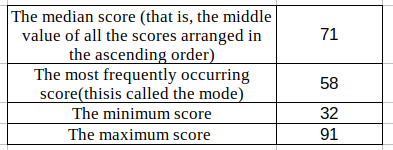There you go – just with these few lines, we can say that a majority of the students performed well, but not many were able to score really high marks in the test. This is what descriptive statistics is. We represented the data of 1000 students using just 5 values.

There are other key terms used in descriptive statistics as well, such as:

• Standard Deviation
• Variance
• Normal distribution
• Central Limit Theorem

### Probability

Based on the same example, let’s say that you are asked a question: if I pick a student randomly from these 1000 students, what are the chances that he/she has passed the test? The concept of probability will help you answer this question. If you get a probability of 0.6, it implies that there is a 60% chance that he/she passed it (assuming the passing criteria is 40 marks).

Other questions on the same data (as shown below) can be answered using Hypothesis testing and Inferential Statistics to answer them:

• Can the entrance test be considered to be tough?
• Was a high score by the student the result of studying hard or because the questions in the test were easy?

You can learn all about statistics and probability from the below resources:

## 5. Key Machine Learning Concepts for Deep Learning

Here’s the good news – you don’t need to know the entire gamut of the Machine Learning algorithms that exist today. Not to say that they are insignificant, but just from the point of view of starting deep learning, there are not many you need to be acquainted with.

There are, however, a few concepts that are crucial to build your foundation and acquaint yourself with. Let us go over these concepts.

### Supervised and Unsupervised algorithms

• Supervised Learning: In these algorithms, we know the target variable (what we want to predict) and we know the input variables (the independent features which contribute to the target variable). We then generate an equation that gives the relationship between the input and the target variables and apply it to the data we have. Examples: kNN, SVM, Linear Regression, etc.
• Unsupervised Learning: In unsupervised learning, we do not know the target variable. It is mainly used to cluster our data into groups, and we can identify the groups after we have clustered the data. Examples of unsupervised learning include k-means clustering, apriori algorithm, etc.

### Evaluation Metrics

Building a predictive model is not the only step required in deep learning. You need to check how good the model is and keep improving it till we reach the best model we can.

So how do we judge the performance of a deep learning model? We use some evaluation metrics. Depending on the task, we have different evaluation metrics for regression and classification.

• Evaluation metrics for Classification:
• Confusion Matrix
• Accuracy
• Precision and Recall
• F1-score
• AUC-ROC
• Log Loss
• Evaluation metrics for Regression:
• RMSE
• RMSLE

Evaluation metrics are extremely crucial in deep learning. Be it in the research domain or in the industry, your deep learning model will be judged on the value of the evaluation metric.

### Validation Techniques

A deep learning model trains itself on the data provided to it. However, as I mentioned above, we need to improve this model and we need to check its performance. The true mettle of the model can only be observed when we give it totally new (although cleaned) data.

But then, how do we improve on the model? Do we give it new data every time we want to change even a single parameter? You can imagine how time-consuming and costly such a task would be!

This is why we use validation. We divide our entire data into 3 parts: training, validation, and testing. Here is a single sentence to help you remember:

We train the model on the training set, improve it on the validation set, and finally predict on the so-far unseen test set.

Some common strategies for Cross-validation are: k-fold Cross-Validation and Leave-One-Out Cross-Validation (LOOCV).

Here’s a comprehensive article covering validation techniques and how to implement them in Python: Improve Your Model Performance using Cross-Validation (in Python / R).

Let us go back to the calculus we saw earlier and the need for optimization. How do we know that we have achieved the best model there can be? We can make small changes in the equation and at each change, we check if we are closer to the actual value.

It is this act of taking small steps towards a possible direction which is the basic intuition behind gradient descent. Gradient descent is one of the most important concepts you will come across and revisit often in deep learning.

Explanation and implementation of Gradient Descent in Python: Introduction to Gradient Descent Algorithm (along with variants) in Machine Learning.

### Linear Models

What is the simplest equation you can think of? Let me list a few:

1. Y = x + 1
2. 4x + 3y -2z = 56
3. Y = x/(1-x)

Did you notice the one thing that was common in all the 3 functions? Yes, they are all linear functions. What if we could predict the value of y using these functions?

These would then be called linear models. You would be surprised to know how popular linear models are in the industry. They are not too complicated, are interpretable, and with the right gradient descent, we can get high evaluation metrics too! Not only this, linear models form the basis of deep learning. For instance, do you know that you can create a logistic regression model using a simple neural network?

Here’s a detailed guide covering not only linear and logistic regression but other linear models as well: 7 Regression Types and Techniques in Data Science.

### Underfitting and Overfitting

You will often come across situations where your deep learning model is performing very well on the training set but gives you poor accuracy on the validation set. This is because the model is learning each and every pattern from the training set, and thus, it is unable to detect these patterns in the validation set. This is called overfitting the data and it makes the model too complex.

On the other hand, if your deep learning model is performing poorly on both the training set as well as the validation set, it is most likely underfitting. Think of it as applying a linear equation (a too simple model) on our data when it is, in fact, non-linear (complex):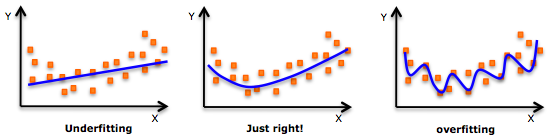A simple analogy for overfitting and underfitting is a student’s example in a math class:

• Overfitting is associated with that student who does rote learning of all the problems discussed in the class but is unable to answer different questions pertaining to the same concepts during the exam
• Underfitting is that student who doesn’t perform well in the class, nor in the exam. We aim for that model/student who need not know all the problems discussed in the class but performs well enough in the exam to show that he/she knows the concepts

Check out this intuitive explanation of overfitting and underfitting, along with the comparison between them: Underfitting vs. Overfitting in Machine Learning.

### Bias-Variance

In simplest terms, bias is the difference between the actual value and the predicted value. Variance is measured by the change in the output when we change the training data.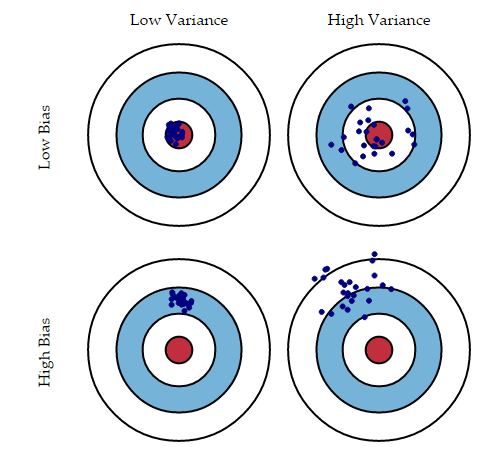Let’s quickly summarize what we can interpret from the above image:

1. Top left: A model that is very accurate, therefore the error of our model will be low, meaning a low bias and low variance. All the data points fit within the bullseye
2. Top right: The predicted data points are centered around the bullseye (low variance), but they are far from each other and from the center as well (high bias)
3. Bottom left: The predicted values are clustered together (low variance), but are pretty far from the bulls-eye (high bias)
4. Bottom right: The predicted data points are neither close to the bullseye (high bias) nor are close to each other (high variance)

Both high bias and high variance lead to an increase in the error. Typically, a high bias implies underfitting, and a high variance implies overfitting. It is very difficult to achieve both low bias and low variance – one usually comes at the cost of the other.

In terms of model complexity, we can use the below diagram to decide on the optimal complexity of our model: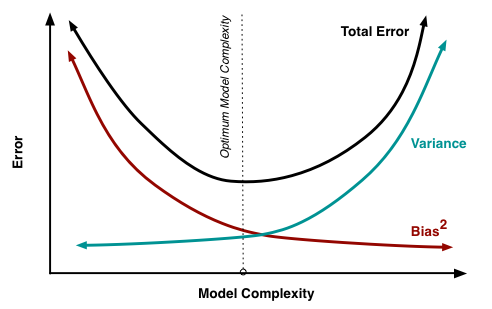I encourage you to go through this awesome essay by Scott Fortmann-Roe on Bias Variance using examples: Understanding the Bias-Variance Tradeoff.

### sklearn

Just like the Pandas library, there is another library that forms the foundation of machine learning. The sklearn library is the most popular library in machine learning. It contains a large number of machine learning algorithms which can you can apply to your data in the form of functions.

What’s more, sklearn even has the functionalities for all the evaluation metrics, cross-validation, and scaling/normalizing your data as well.

Here’s a quick example of sklearn in action:

```from sklearn import datasets, linear_model
from sklearn.metrics import mean_squared_error

regr = LinearRegression()

#train your data - remember how we train the model on our train set?
regr.fit(X_train, y_train)

#predict on our validation set to improve it
y_pred = regr.predict(X_Valid)

#evaluation metrics: MSE
print('Mean Squared Error:', mean_squared_error(y_test, y_pred))
...#further improvement of our model```

There you go! We could build a simple linear regression model with essentially less than 10 lines of code!

## End Notes

In this article, we covered 5 essential things you need to know before building your first deep learning model. It is here that you will encounter the popular deep learning frameworks like PyTorch and TensorFlow. They have been built with Python in mind and you can now easily understand working with them since you have a good grasp of Python.

Here are a couple of great articles to get started on these frameworks:

Once you have built your foundations on these 5 pillars, you can always explore more advanced concepts like Hyperparameter Tuning, Backpropagation, etc. These are the concepts I built my knowledge of deep learning on.###### Purva Huilgol